# 线性方程

### 以下的都是线性方程：

 y = 2x + 1 5x = 6 + 3y y/2 = 3 − x

### 例子：y = 2x + 1是线性方程：y = 2x+1 的图是一条直线

• 当 x 增加时，y 以 双倍的速度增加，所以我们需要 2x
• 当 x 是 0，y 已经是 1，所以也需要 +1
• 所以：y = 2x + 1

x y = 2x + 1
-1 y = 2 × (-1) + 1 = -1
0 y = 2 × 0 + 1 = 1
1 y = 2 × 1 + 1 = 3
2 y = 2 × 2 + 1 = 5

## 不同形式

### 例子：这些都是线性方程：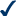y = 3x − 6y − 2 = 3(x + 1)y + 2x − 2 = 05x = 6y/2 = 3

### 例子：这些都不是线性方程：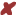y2 − 2 = 03√x − y = 6x3/2 = 16

## 斜截式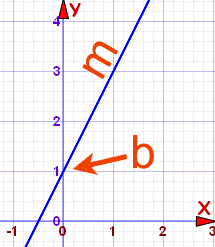坡度 （或 斜率）) Y截距

### 例子：y = 2x + 1

（页顶的例子，为斜截式）

• 坡度：m = 2
• 截距： b = 1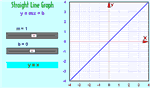## 点斜式

 y − y1 = m(x − x1)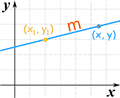• x1 = 2
• y1 = 3
• m = ¼

## 一般式

 Ax + By + C = 0 （A 和 B 不能两者都等于 0）

• A = 3
• B = 2
• C = −4

## 函数式

 y = 2x − 3 f(x) = 2x − 3 这些是一样的！

 y = 2x − 3 w(u) = 2u − 3 h(z) = 2z − 3 这些也是一样的！

## 恒等函数

f(x) = x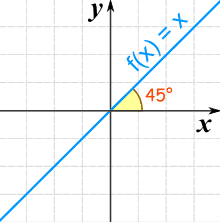0 0
5 5
−2 −2
。。。。。。等等 。。。。。。等等

## 常数函数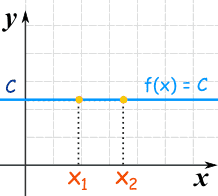f(x) = C